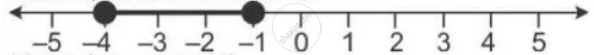Share

# Given a = {X: -1 < X ≤ 5, X ∈ R} and B = {X: -4 ≤ X < 3, X ∈ R} Represent on Different Number Lines: A' ∩ B - Mathematics

Course

#### Question

Given A = {x: -1 < x ≤ 5, x ∈ R} and B = {x: -4 ≤ x < 3, x ∈ R}
Represent on different number lines:

A' ∩ B

#### Solution

Numbers which belong to B but do not belong to A = B - A

A' ∩ B = {x : -4 <= x <= -1 , x ∈ R}

It can be represented on a number line asIs there an error in this question or solution?

#### APPEARS IN

Selina Solution for Concise Mathematics for Class 10 ICSE (2020 (Latest))
Chapter 4: Linear Inequations (In one variable)
Exercise 4(B) | Q: 17.2 | Page no. 50
Solution Given a = {X: -1 < X ≤ 5, X ∈ R} and B = {X: -4 ≤ X < 3, X ∈ R} Represent on Different Number Lines: A' ∩ B Concept: Representation of Solution on the Number Line.
S